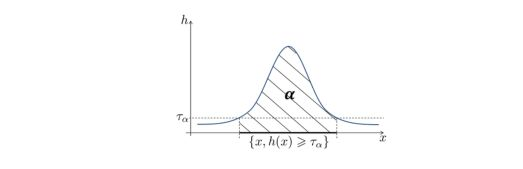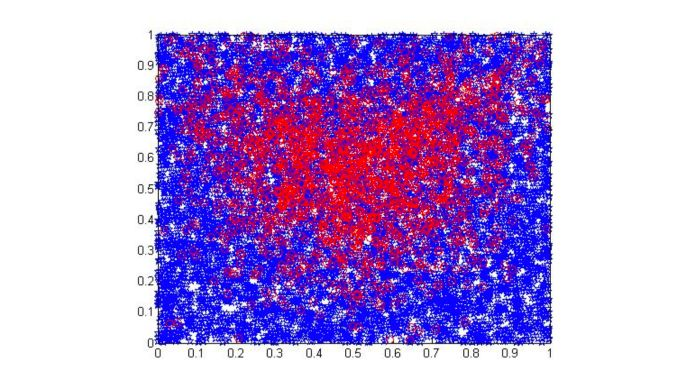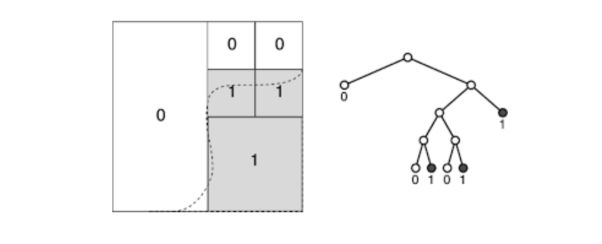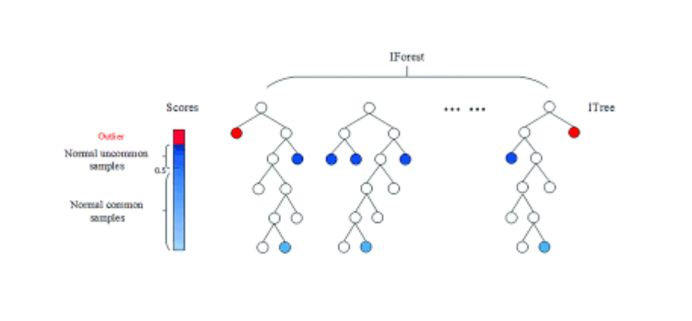“An anomaly is an observation that deviates so much from other observations as to arouse suspicions that it was generated by a different mechanism.”, Hawkins (1980)

Anomaly detection is used in :

• network intrusions
• creit card fraud detection
• insurance
• finance
• surveillance
• rental services

There are 3 types of anomaly detection :

• supervised : we have labels for both normal data and anomalies
• semi-supervised : only normal data is available, no outliers are present
• unsupervised : no labels, we suppose that anomalies are rare events

The key steps in anomaly detection are the following :

• learn a profile of a normal behavior, e.g. patterns, summary statistics…
• use that normal profile to build a decision function
• detect anomalies among new observations

# Unsupervised Anomaly Detection

In unsupervised anomaly detection, we make the assumption that anomalies are rare events. The underlying data are unlabeled (no normal/abnormal label), hence the denomination. Anomalies are events supposed to be locates in the tail of the distribution.

## Minimum Volume Set

We usually estimate the region where the data is the most concentrated as the minimal region containing $$\alpha %$$ of the values. This approach is called Minimum Volume Set. Samples that do not belong to the Minimum Volume Set are anomalies. For a small value of $$\alpha$$ on the other hand, we tend to recover the modes.

We can define the MV Set as :

$Q( \alpha ) = argmin_{c \in C} \{ \lambda(C), P(X \in C) ≥ \alpha \}$

Where :

• $$\alpha$$ is a factor close to 1 that reflects the percentage of values in the MV Set.
• $$C$$ classes of measurable sets
• $$\lambda$$ a Lebesgue measure
• $$\mu(dx)$$ the unknown probability measure of the observations

The goal is to learn a minimum volume set $$Q(\alpha)$$ for $$X_1, \cdots, X_n$$There are theoretical guarantees that there exists a unique MV set at level $$\alpha$$.

Let’s now make the assumption that $$\mu$$ has a density $$h(X)$$ that :

• is bounded
• has no plateau

## Algorithms for anomaly detection

### Plug-in technique

This technique consists in seeing MV sets as density level sets :

$G_{\alpha}* = \{ x \in R^d : h(x) ≥ t_{\alpha} \}$

Using a naive approach (the 2-split trick), we can :

• compute a density estimator using parametric models or local averaging $$\hat{h}(x)$$ based on $$X_1, \cdots, X_n$$
• based on a second sample $$X_1*, \cdots, X_n*$$, compute the empirical quantile corresponding to the $$\alpha$$ percentile

The output is :

$\hat{G_{\alpha}} * = \{ x: \hat{h_n(x)} ≥ \hat{h_n} ( X_{n \alpha}' ) \}$

### Unsupervised as binary classification

We can turn the unsupervised anomaly detection problem as a binary classification.

• Suppose that our data lie into $$[0,1]^d$$, or that we scaled the data before. We have $$n$$ points.
• We then generate a new sample from the uniform distribution $$U([0,1]^d)$$. We generate $$m$$ points.
• We assign a negative label to the generated sample.
• We assign a positive label to the true sample.

We have :

$$\frac{n}{n+m} = p$$ approximately.The solution of this binary classification mimics the Bayes Classifier where we predict $$1$$ on the set $$\{ x \in [0,1]^d : h(x) ≥ \frac{1-p}{p} \}$$, and $$-1$$ otherwise.

This solution provides a MV set at level $$\alpha = P(h(X) ≥ \frac{1}{p-1})$$. We select $$\alpha$$ manually, and we tune $$p$$ using a grid search.

### Histograms

In histograms, we suppose that we have a compact feature space, and that we build partitions $$C_1, \cdots, C_K$$ formed of measurable subsets of same volume :

$\lambda(C_1) = \cdots = \lambda(C_K)$

The class $$G_P$$ is the ensemble composed of unions of $$C_K$$. We want to get the minimal number of partitions to keep in order to isolate the normal data and exclude the anomalies. More formally, we are looking for the solution of :

$\min_{G \in P : \hat{\mu_n}(G) ≥ \alpha - \phi} \lambda(G)$

There is a simple and fast procedure to do this :

• For each class $$k = 1 \cdots K$$, we compute $$\hat{\mu_n}(C_k)$$, i.e the proportion of data in each class
• Sort the cells by decresing order of $$\hat{\mu_n}(C_k)$$
• Find the minimal value of k such that : $$\hat{k} = argmin \{ k : \sum_i \hat{\mu_n}(C_i) ≥ \alpha - \phi \}$$. In other words, we exclude $$\alpha$$ percent of the values based on this histogram.
• The output is $$\hat{G_{\alpha}}* = U_i^{\hat{k}} C_{(i)}$$

Where $$\phi$$ is a tolerance level. However, such technique is usually not flexible enough.

### Decision Trees

The partition should be determined based on the data. We adopt a top-down strategy, and start from the root node $$C_{0,0}$$. The volume of any cell $$C_{j,k}$$ can be computed in a recursive manner.Hyperrectangless with the label 1 are subsets of the MV set estimate. At each node $$C_{j,k}$$, the split leading to siblings $$C_{j+1,2k}$$ and $$C_{j+1,2k+1}$$ minimizes recursively the volume of the current ddecision set G under the following constraint :

$\hat{\mu_n}(G) ≥ \alpha(-\phi)$

To avoid overfitting, we generally prune the tree after growing it to avoid overfitting.

### Isolation Forest

In insolation forests, we build a forest of isolation trees under the assumption that anomalies are more susceptible to isolation under random partitioning.

The main idea behind an isolation tree is to recursively (top-down) build a tree that :

• chooses randomly a splitting variable
• splits at value $$t$$
• splits the cells if the obsevation is larger or lower than $$t$$

This process stops when a depth limit is reached usually :# Supervised Anomaly Detection

In the supervised Setup, we have pairs of data $$(X,Y)$$ values in $$R^d \times \{-1,+1\}$$. If a data point has a positive label (Y=1), it is an anomaly. This is a classic binary classification task.

We also define a randking among anomalies, with the first data points the ones that have the highest probability to be anomalies. This is called Bipartite Ranking. It is a different approach from classification, since classification remains a local task, whereas ranking is global.

We rank and score a set of instances through a scoring function $$s(X)$$ in which large number instances with label 1 appear on top of the list with high probabilities.

How do we measure the quality of a ranking?

A natural estimate of the ranking performance :

$U(s) = P \{ (s(X) -s(X'))(Y-Y')>0\}$

is the U-Statistic :

$\hat{U}_n(s) = \frac{2}{n(n-1)} \sum_{1≤i<j≤n}1 \{ (s(X_i) - s(X_j))(Y_i - Y_j) > 0 \}$

This measure is also called the rate of concording pairs, or Kendall’s association coefficient. One of the issues is that the computation of the gradients typically require to average over $$O(n^2)$$ pairs.

The criterion we want to maximize is :

$L(s) = P \{s(X^{(1)}) < \cdots < s(X^{(k)}) \mid Y^{(1)} = 1, \cdots, Y^{(k)} = K \}$

The empirical counterpart of $$L(s)$$ is really prohibitive to compute, and the maximization is even unfeasible. To overcome such issues, generalized U-Statistics using Kernels or incomplete U-Statistics using only a sample of terms can be used.

Categories:

Updated: